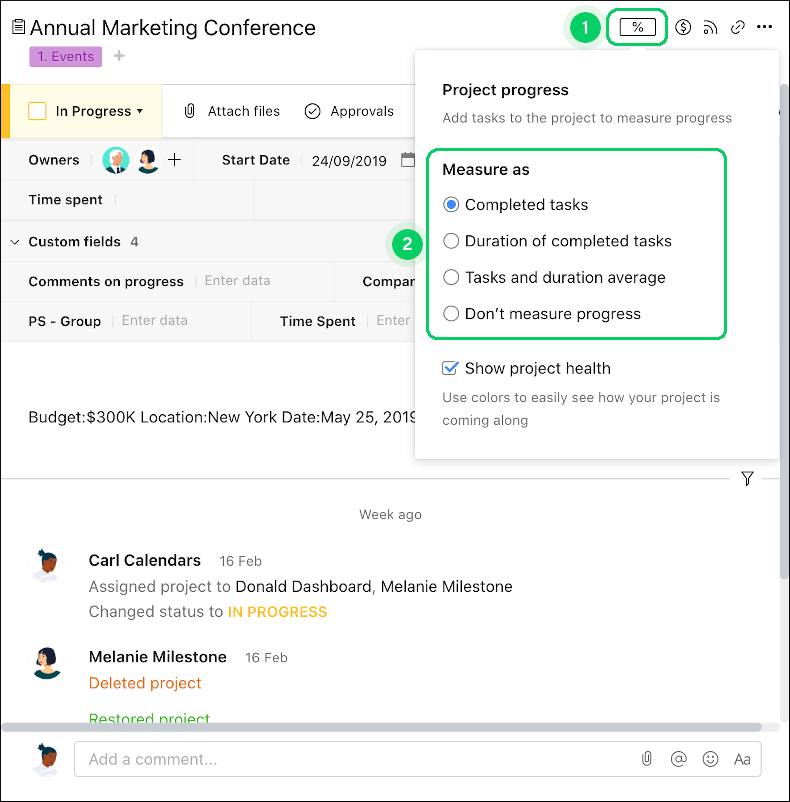All articles

# Project Progress Metrics

Table 66. Availability

## Overview

Project progress is available on Business and Enterprise accounts. All users, including collaborators, can see project progress.

Project progress allows Wrike to automatically calculate if your projects are on track once it's enabled. You can select one of three metrics to calculate it.

Keep in mind:

• Tasks that live in a project’s subfolders and subprojects are taken into account when the project progress is calculated.

• Deferred and canceled tasks don’t affect project progress.

• You may have different progress metrics enabled in your Android app and Wrike web version. Therefore, progress values may differ.

## How project progress is calculated

You can select one of three metrics for how Wrike will calculate your project progress.

Table 67. Project Progress Metrics

Metric

How it’s calculated

Example

The number of completed tasks is divided by the total number of tasks, and the result is multiplied by 100%.

• Three of them are completed.

Progress by number is calculated as:

(3 / 10) * 100% = 30%

The duration of completed tasks is divided by the total duration of tasks in the project and the result is multiplied by 100%.

If the duration of a project’s tasks is 0 days or not specified, this progress metric based on duration is calculated as 100%.

• Their durations are 1, 2, and 5 days respectively.

• The total duration of tasks is 1 + 2 + 5 = 8

Progress by duration is calculated as:

2 days (duration of completed tasks) / 8 days (total duration) * 100% = 25%

The two metrics listed above are averaged.

• Completed tasks are calculated to be 30%

• Duration of completed tasks is calculated to be 40%.

Tasks and duration average is calculated as:

(30% + 40%) / 2 = 35%

## Select a project progress metric

1. Navigate to the relevant project and open its project info panel.

2. Click the project progress icon in the top-right corner 1.

3. Select Completed tasks, Duration of completed tasks, or Tasks and duration average under Measure as 2.

4. Your project progress is shown or updated as a percentage based on your choice.Top